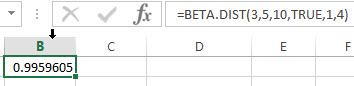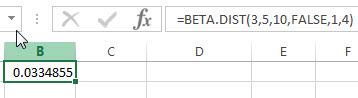# Excel BETA.DIST Function

This post will guide you how to use the BETA.DIST function with syntax and examples in Microsoft excel.

### Description

The Excel BETA.DIST function returns the beta distribution. So you can use the BETA.DIST function to calculate the cumulative beta distribution for a given set of parameters in Excel. And the beta distribution is used to study variation in the percentage of something across samples.

The BETA.DIST function is a build-in function in Microsoft Excel and it is categorized as a Statistical Function.

The BETA.DIST function is available in Excel 2016, Excel 2013, Excel 2010, Excel 2011 for Mac.

### Syntax

The syntax of the BETA.DIST function is as below:

`= BETA.DIST(x, alpha, beta, cumulative, [A], [B])`

Where the BETA.DIST function arguments are:

• X -This is a required argument.  The value that is between A and B at which the function is to be calculated.
• Alpha – This is a required argument.  It is a parameter of the distribution.
• Beta – This is a required argument.  It is a parameter of the distribution.
• Cumulative – This is a required argument. It is a logical value that specifies the type of distribution to be used. If the cumulative is TRUE, the BETA.DIST function returns the cumulative distribution function; if it is equal to FALSE, the BETA.DIST function returns the probability density function.
• A – This is an optional argument. A lower bound to the interval of x. If it is omitted, it will be set the default value 0.
• B – This is an optional argument. A upper bound to the interval of x. If it is omitted, it will be set the default value 1.

Note:

• If any argument is non-numeric, the BETA.DIST function will return the #VALUE! Error.
• If the Alpha or Beta argument is smaller than or equal to 0, then the BETA.DIST function will return #NUM! Error.
• If the argument X is small than A and greater than B, the BETA.DIST function will Return #NUM! Error.

### Excel BETA.DIST Function Examples

The below examples will show you how to use Excel BETA.DIST Function to calculate the cumulative beta distribution or beta probability density function.

1# to calculate the cumulative beta probability density function for a supplied set of parameters, using the following formula:

`=BETA.DIST(3,5,10,TRUE,1,4)`2# to calculate beta probability density function for a supplied set of parameters, using the following formula:

`=BETA.DIST(3,5,10,FALSE,1,4)`